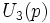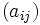Difference between revisions of "Unitriangular matrix group:UT(3,p)"

This article is about a family of groups with a parameter that is prime. For any fixed value of the prime, we get a particular group.
View other such prime-parametrized groups

Definition

Given a prime$p$, the group$U_3(p)$ is defined as follows: it is the group of upper triangular matrices with 1s on the diagonal, and entries over$F_p$ (with the group operation being matrix multiplication).

Each such matrix$(a_{ij})$ can be described by the three entries$a_{12}, a_{13}, a_{23}$. The multiplication of matrices$A = (a_{ij})$ and$B = (b_{ij})$ gives the matrix$C = (c_{ij}$ where:

•$c_{12} = a_{12} + b_{12}$
•$c_{13} = a_{13} + b_{13} + a_{12}b_{23}$
•$c_{23} = a_{23} + b_{23}$

Elements

The analysis given below does not apply to the case$p = 2$. For$p = 2$, we get the dihedral group:D8, which is studied separately.

Upto conjugacy

Every element has order$p$.

The center has order precisely$p$, so there are$p$ elements that form conjugacy classes of size 1. These are, specifically, the elements with$a_{12} = a_{23} = 0$, and they're thus parametrized by their$a_{13}$ entry.

For every element outside the center, the centralizer of that element is the subgroup generated by that element and the center, and is hence of order$p^2$. Thus, the conjugacy class of the element is of size$p$.

Thus, there is a total of$p$ conjugacy classes of size 1 and$p^2 - 1$ conjugacy classes of size$p$.

Upto automorphism

There are only three classes of elements upto automorphism:

1. The identity element, which forms a class of size 1
2. The non-identity elements in the center, which form a class of size$p - 1$
3. The non-central elements, which form a class of size$p^3 - p$

Subgroups

Here is the complete list of subgroups:

1. The trivial subgroup (1)
2. The center, which is a group of order$p$. In matrix terms, this is the subgroup comprising matrices$a_{ij}$ with$a_{12} = a_{23} = 0$. (1)
3. Subgroups of order$p$ generated by non-central elements. These are not normal, and occur in conjugacy classes of size$p$. ($p(p+1)$)
4. Subgroups of order$p^2$ containing the center. These are the inverse images via the quotient map by the center, of subgroups of order$p$ in the inner automorphism group. ($p + 1$)
5. The whole group. (1)

Normal subgroups

The subgroups in (1), (2), (4) and (5) above are normal.

Characteristic subgroups

The subgroups in (1), (2) and (5) above are normal. In other words, there are only three characteristic subgroups. Some notable facts:

Fully characteristic subgroups

The fully characteristic subgroups are precisely the same as the characteristic ones.

The central factors are precisely the same as the characteristic subgroups.

There are no proper nontrivial direct factors. In other words, the group is directly indecomposable.

Endomorphisms

Automorphisms

The automorphisms essentially permute the subgroups of order$p^2$ containing the center, while leaving the center itself unmoved.

Related groups

For any prime$p$, there are two non-Abelian groups of order$p^3$. One of them is this, and the other is the semidirect product of the cyclic group of order$p^2$ by a group of order$p$ acting by power maps (with the generator corresponding to exponentiation by$p+1$.